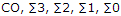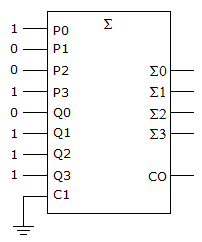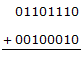# Digital Electronics - Digital Arithmetic Operations and Circuits

### Exercise :: Digital Arithmetic Operations and Circuits - General Questions

66.

Subtract the following hexadecimal numbers.
 47 34 FA –25 –1C –2F

 A. 22    18    CB B. 22    17    CB C. 22    19    CB D. 22    18    CC

Answer: Option A

Explanation:

No answer description available for this question. Let us discuss.

67.

What is the correct output of the adder in the given figure, with the outputs in the order:A. 10111 B. 11101 C. 01101 D. 10011

Answer: Option A

Explanation:

No answer description available for this question. Let us discuss.

68.

Solve this binary problem:A. 11001001 B. 10010000 C. 01101110 D. 01110110

Answer: Option B

Explanation:

No answer description available for this question. Let us discuss.

69.

The BCD addition of 910 and 710 will give initial code groups of 1001 + 0111. Addition of these groups generates a carry to the next higher position. The correct solution to this problem would be to:

 A. ignore the lowest order code group because 0000 is a valid code group and prefix the carry with three zeros B. add 0110 to both code groups to validate the carry from the lowest order code group C. disregard the carry and add 0110 to the lowest order code group D. add 0110 to the lowest order code group because a carry was generated and then prefix the carry with three zeros

Answer: Option D

Explanation:

No answer description available for this question. Let us discuss.

70.

Subtract the following binary numbers.
 0101 1000 1010 0011 1101 1110 –0010 0011 –0011 1000 –0101 0111

 A. 0011  0100    0110  1010    1000  0110 B. 0011  0101    0110  1011    1000  0111 C. 0011  0101    0110  1010    1000  0111 D. 0011  0101    0110  1010    1000  0110

Answer: Option B

Explanation:

No answer description available for this question. Let us discuss.

#### Current Affairs 2021

Interview Questions and Answers